Learn all Concepts of Polynomials Class 9 (with VIDEOS). Check - Polynomials Class 9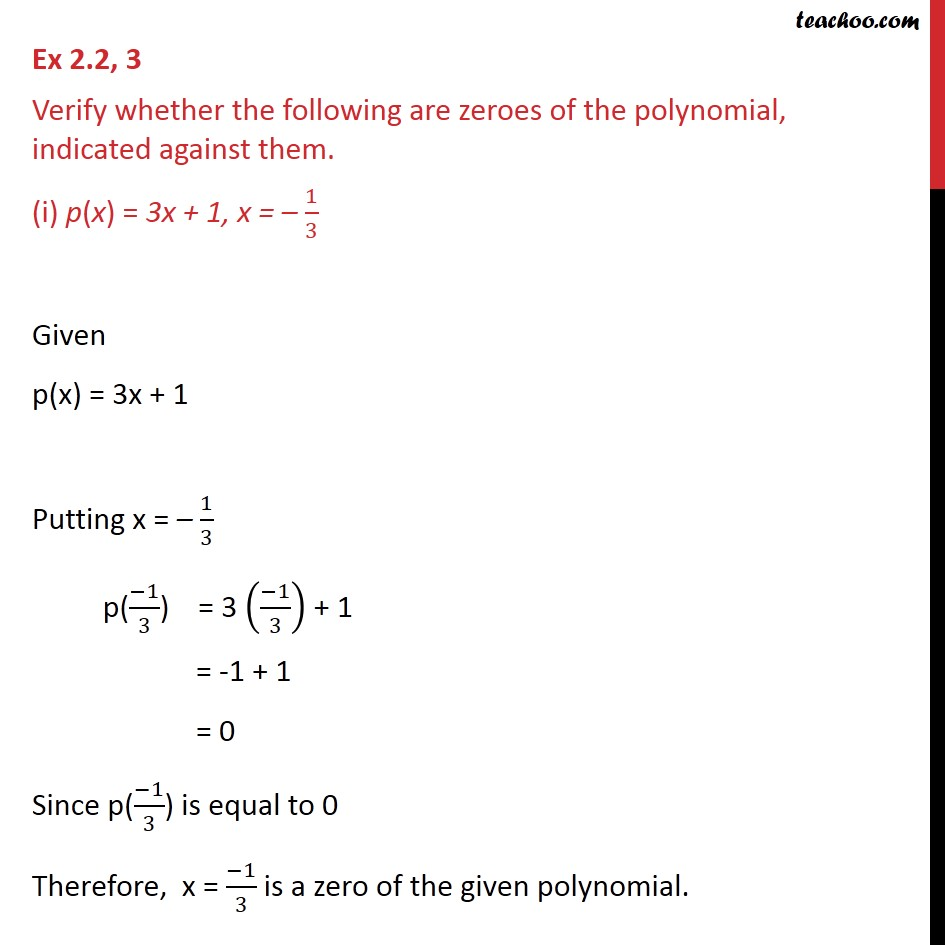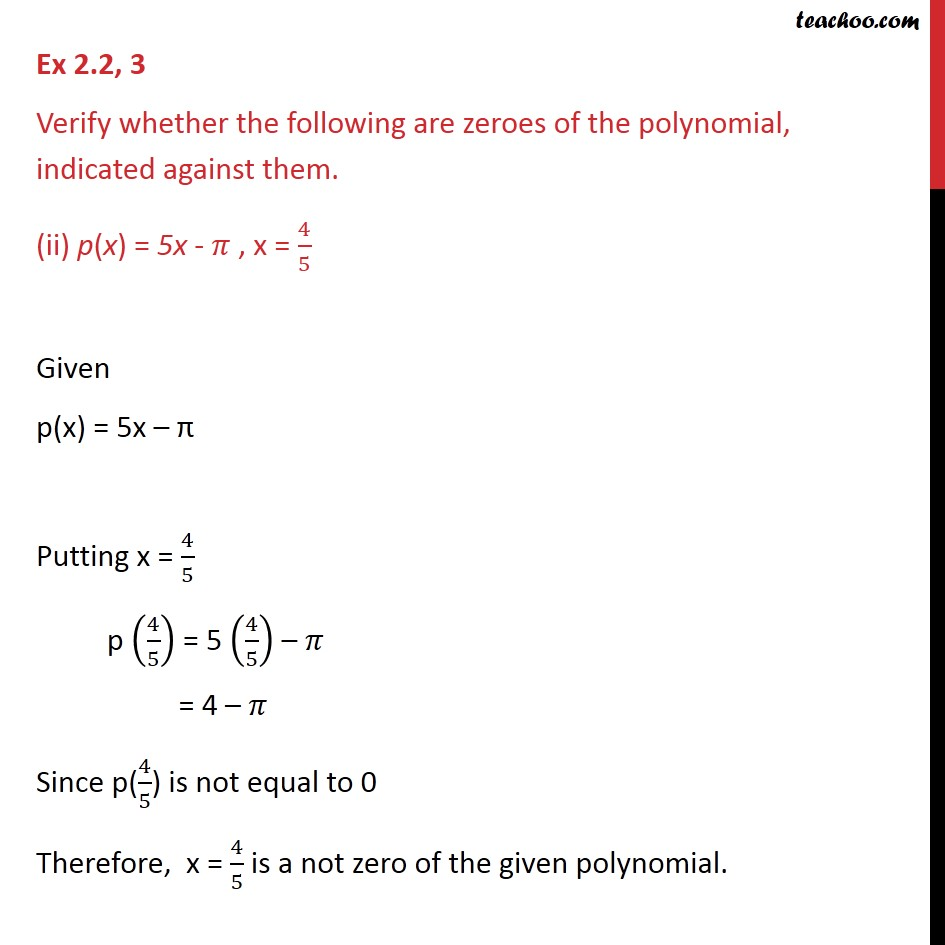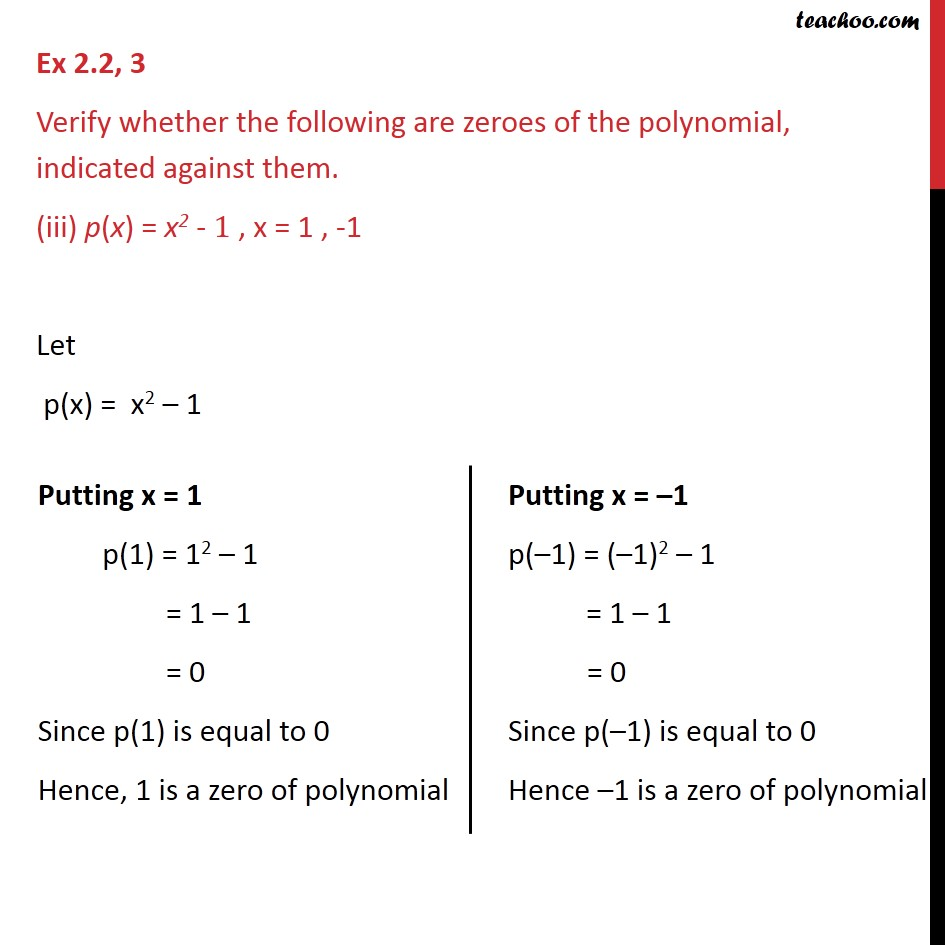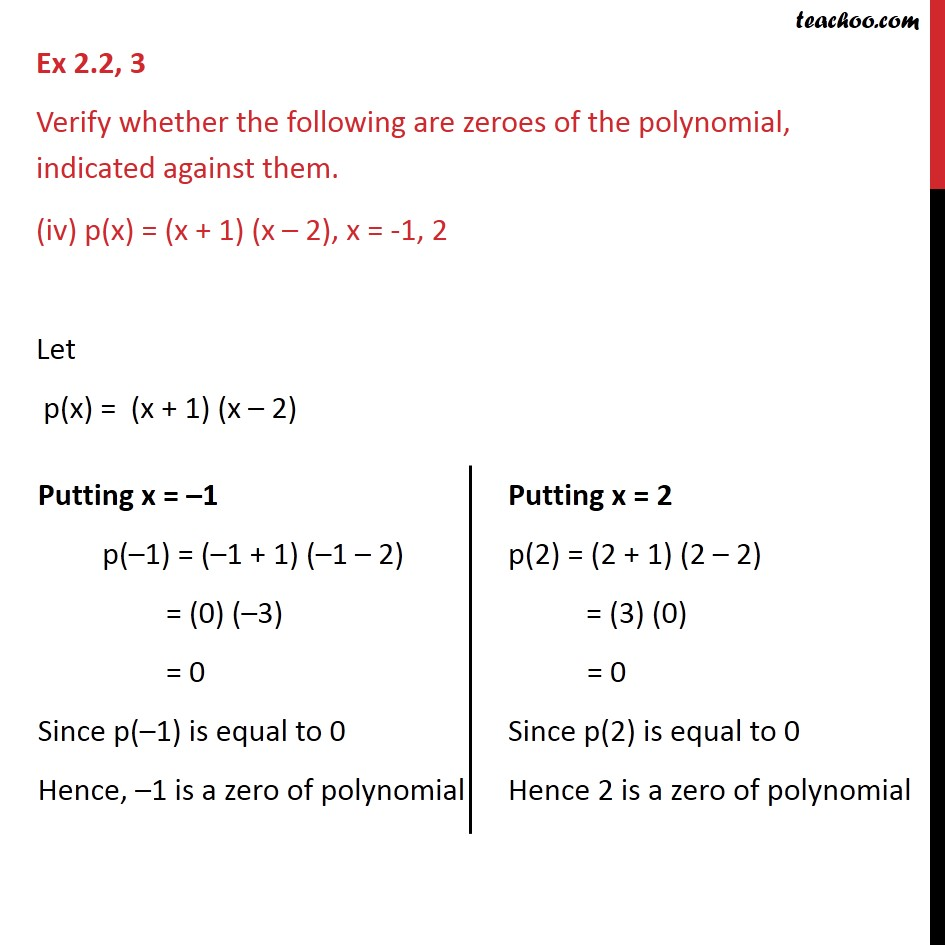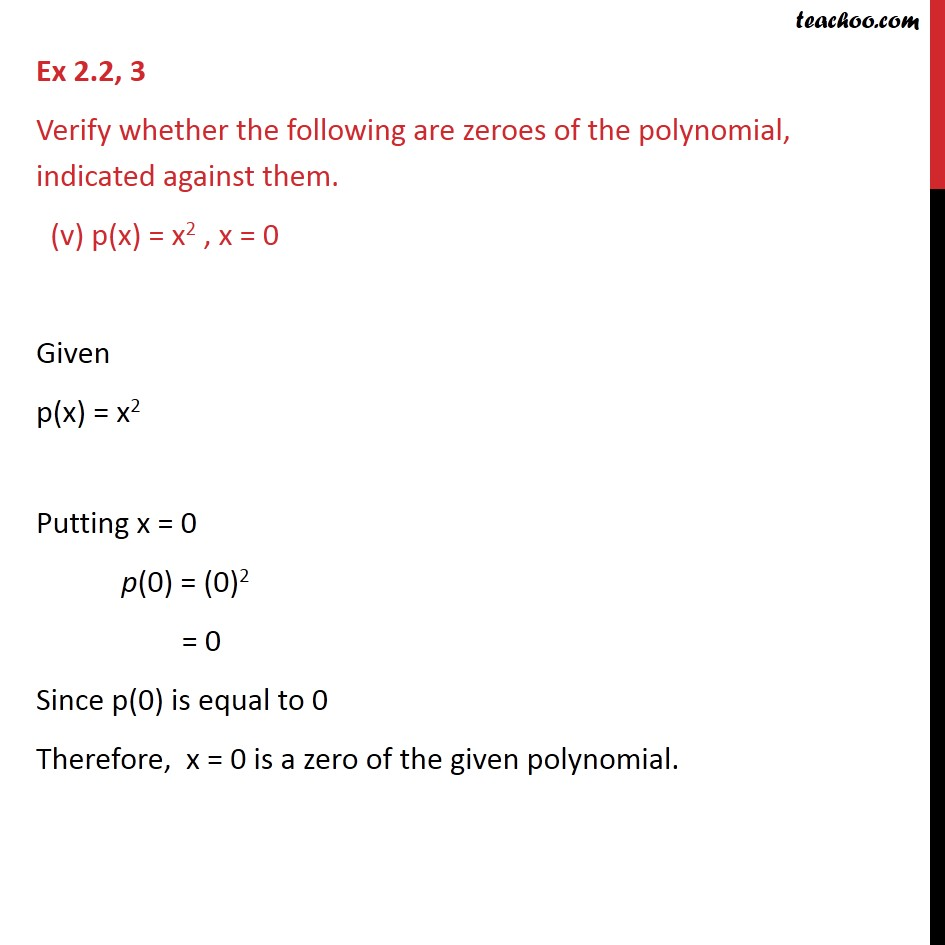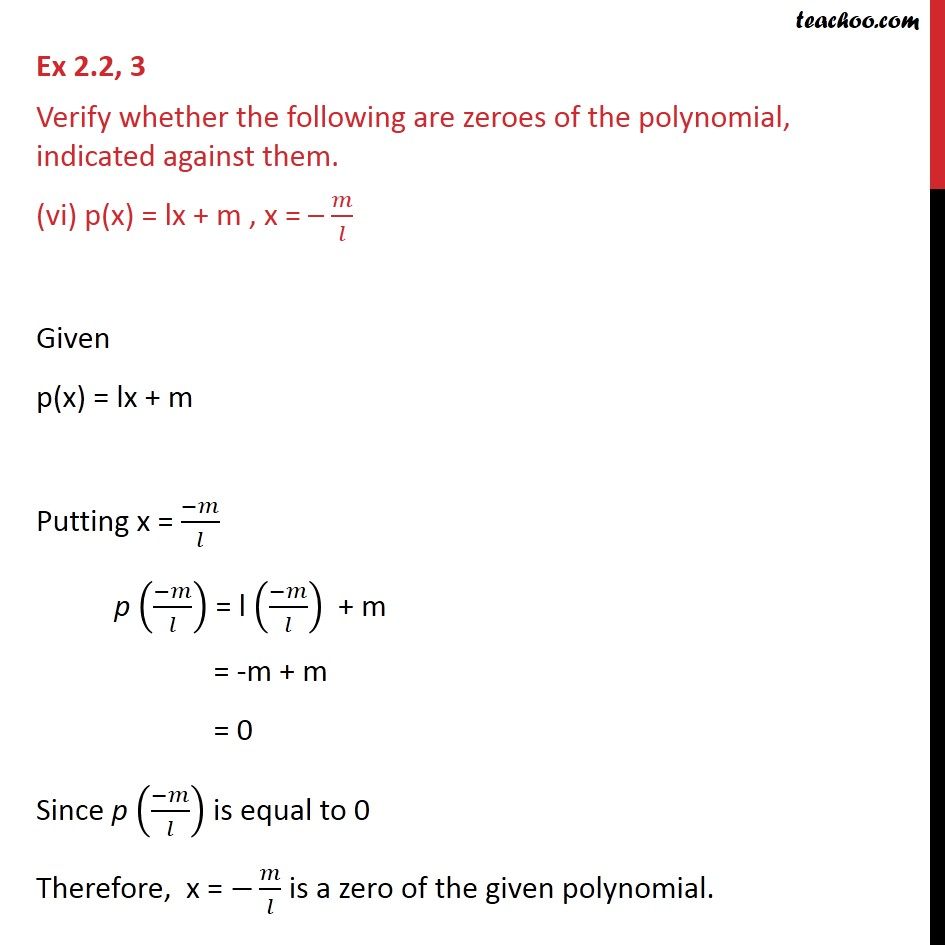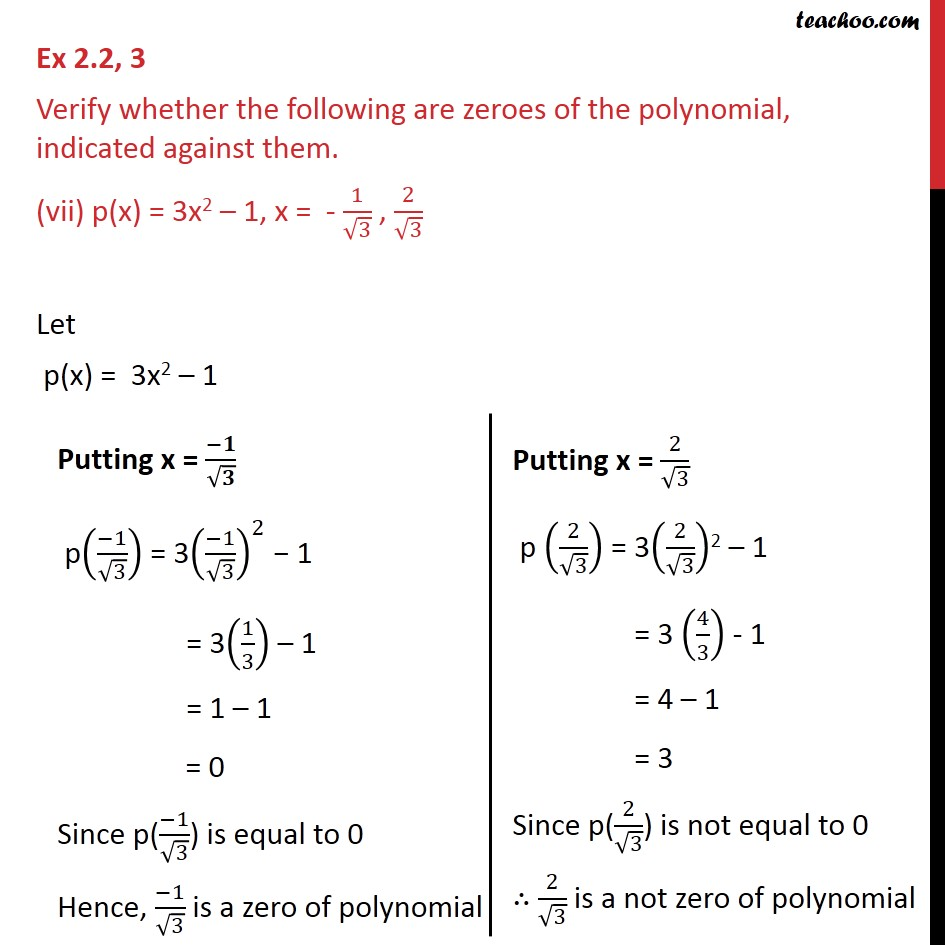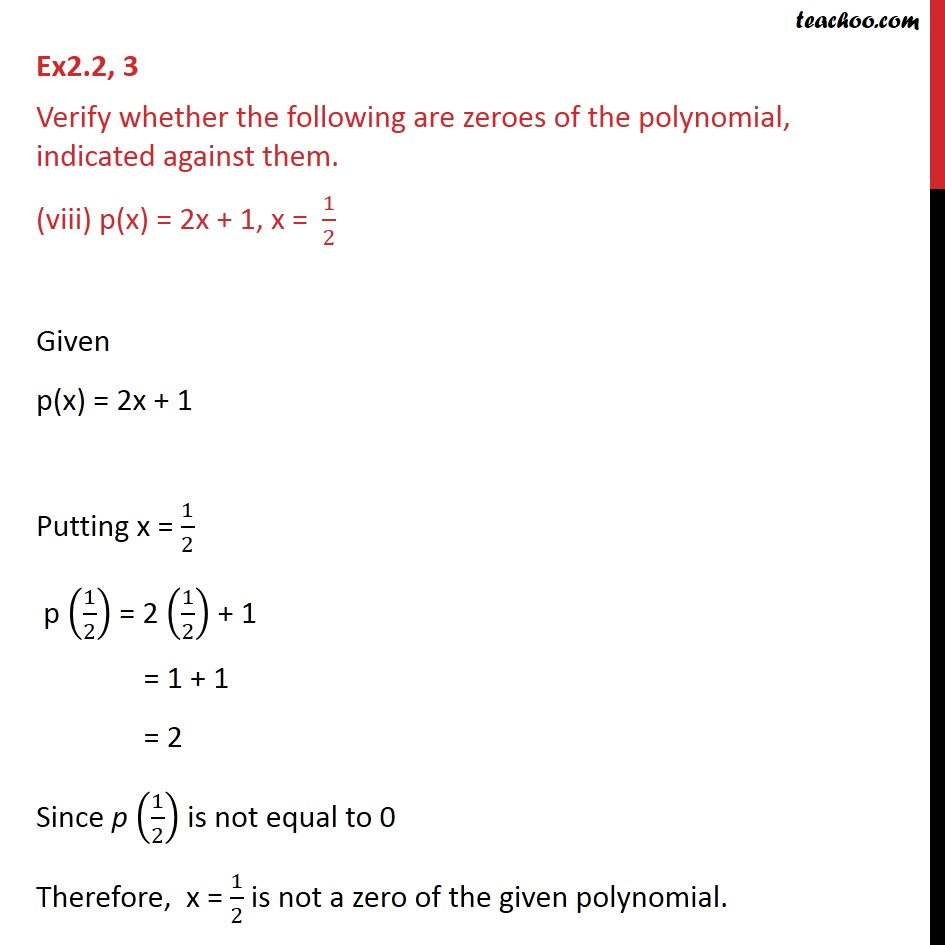1. Chapter 2 Class 9 Polynomials
2. Serial order wise
3. Ex 2.2

Transcript

Ex 2.2, 3 Verify whether the following are zeroes of the polynomial, indicated against them. (i) p(x) = 3x + 1, x = – 1/3 Given p(x) = 3x + 1 Putting x = – 1/3 p((−1)/3) = 3 ((−1)/3) + 1 = -1 + 1 = 0 Since p((−1)/3) is equal to 0 Therefore, x = (−1)/3 is a zero of the given polynomial. Ex 2.2, 3 Verify whether the following are zeroes of the polynomial, indicated against them. (ii) p(x) = 5x - 𝜋 , x = 4/5 Given p(x) = 5x – π Putting x = 4/5 p (4/5) = 5 (4/5) – 𝜋 = 4 – 𝜋 Since p(4/5) is not equal to 0 Therefore, x = 4/5 is a not zero of the given polynomial. Ex 2.2, 3 Verify whether the following are zeroes of the polynomial, indicated against them. (iii) p(x) = x2 - 1 , x = 1 , -1 Let p(x) = x2 – 1 Ex 2.2, 3 Verify whether the following are zeroes of the polynomial, indicated against them. (iv) p(x) = (x + 1) (x – 2), x = -1, 2 Let p(x) = (x + 1) (x – 2) Ex 2.2, 3 Verify whether the following are zeroes of the polynomial, indicated against them. (v) p(x) = x2 , x = 0 Given p(x) = x2 Putting x = 0 p(0) = (0)2 = 0 Since p(0) is equal to 0 Therefore, x = 0 is a zero of the given polynomial. Ex 2.2, 3 Verify whether the following are zeroes of the polynomial, indicated against them. (vi) p(x) = lx + m , x = – 𝑚/𝑙 Given p(x) = lx + m Putting x = (−𝑚)/𝑙 p ((−𝑚)/𝑙) = l ((−𝑚)/𝑙) + m = -m + m = 0 Since p ((−𝑚)/𝑙) is equal to 0 Therefore, x = −𝑚/𝑙 is a zero of the given polynomial. Ex 2.2, 3 Verify whether the following are zeroes of the polynomial, indicated against them. (vii) p(x) = 3x2 – 1, x = - 1/√3 , 2/√3 Let p(x) = 3x2 – 1 Ex2.2, 3 Verify whether the following are zeroes of the polynomial, indicated against them. (viii) p(x) = 2x + 1, x = 1/2 Given p(x) = 2x + 1 Putting x = 1/2 p (1/2) = 2 (1/2) + 1 = 1 + 1 = 2 Since p (1/2) is not equal to 0 Therefore, x = 1/2 is not a zero of the given polynomial.

Ex 2.2

Chapter 2 Class 9 Polynomials
Serial order wise